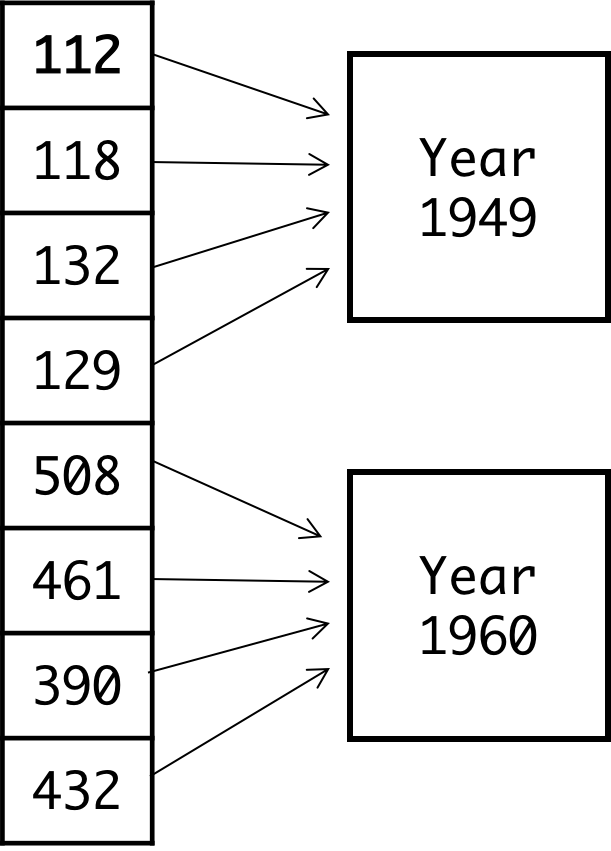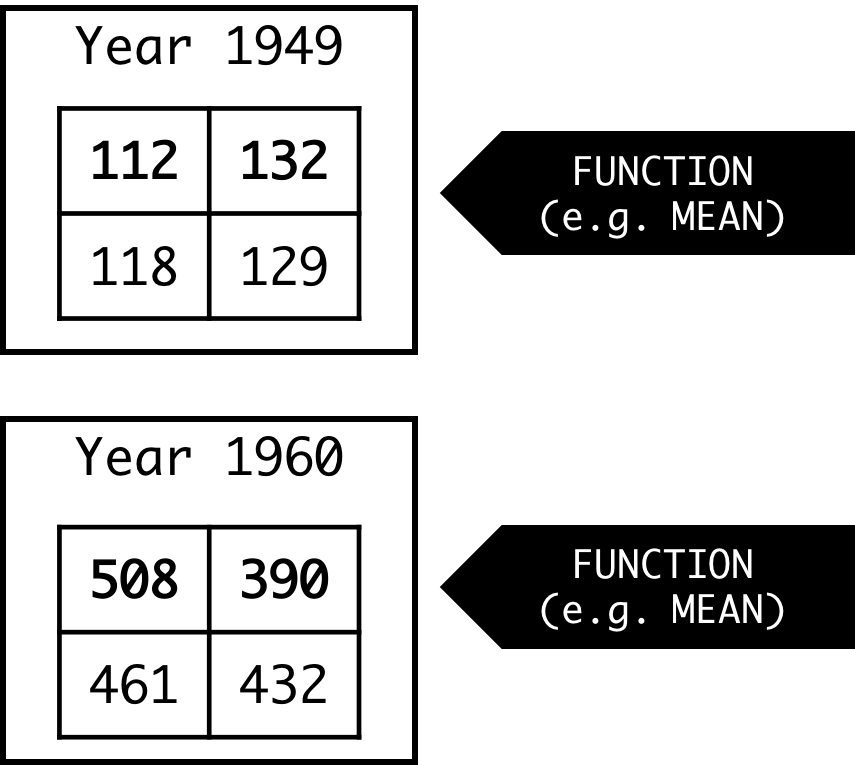Load the restructured data set AirPassengers (see help(AirPassengers)) in R:

load('dat-AirPassengers.rda')

## AGGREGATING DATA

Instead of plotting each trial, we are generally interested in visualizing the grand averages, i.e. the means per condition over time. There are different function in R that facilitate in calculating averages per condition, such as aggregate, tapply, and ddply.

These three functions work roughly in the same way. They expect three arguments:

1. the data to be aggregated, for example a column with proportions or empirical logits;

2. a list or vector (depending on the function) with vectors that specify the conditions or groups to split the data;

3. a function to apply to the data for each condition or group.

Consider the following data, which includes 8 measurements (the first and last 4 data points of the data set AirPassengers):

smalldat
##   AirPassengers Month Year
## 1           112     1 1949
## 2           118     2 1949
## 3           132     3 1949
## 4           129     4 1949
## 5           508     9 1960
## 6           461    10 1960
## 7           390    11 1960
## 8           432    12 1960

We would like to calculate the mean number of air passengers per year. The functions tapply, aggregate, and ddply (all explained in more detail below) first split the data column Passengers into groups define by the column Year:After that, a function such as the mean (or any other function, e.g. min or length) will be applied to each group.This is the basic idea behind the aggregation functions in R, but they all use different syntax and have a different output structure. Below we provide examples for all three functions, to highlight the differences. (Here you could download the data frame and try the functions yourself.)

#### tapply

The function tapply outputs a table rather than a data frame. The columns that specify the groups are listed in a list:

test <- tapply(dat$AirPassengers, list(dat$Year), mean)
# or - less typing:
test <- with(dat, tapply(AirPassengers, list(Year), mean) )
test 

Instead of the average (mean), we could also use another function, such as the standard deviation (sd), the number of observations (length), or even specify our own function.

test <- tapply(dat$AirPassengers, list(dat$Year), length)

#### aggregate

The function aggregate is very similar to the function tapply, but it outputs a data.frame.

test <- aggregate(dat$AirPassengers, list(dat$Year), mean)
test

Note that in this example, the column names are not meaningful: the data column is called x and the grouping predictor is labeled as Group.1 To add column names, we could add these names explicitly to the vectors wrapped in a list. Alternatively, aggregate allows for a formula-input. Both methods are illustrated below.

# Method 1:
test <- aggregate(list(AirPassengers=dat$AirPassengers), list(Year=dat$Year), mean)
test
# Method 2:
test <- aggregate(AirPassengers ~ Year, data=dat, mean)
test

#### ddply

Finally, a third method to calculate averages is by using the function ddply from the plyr package. (See preparations of Lab 1 on how to install an R package.) This function outputs a data frame, like the function aggregate is doing too. The advantage of ddply is that it also to calculate multiple measures for the same groups. Here’s an example:

## if you need to install the plyr package:
# install.packages("plyr")

# load library
library(plyr)
test <- ddply(dat, c("Year"), summarise,
avg = mean(AirPassengers),
n    = length(AirPassengers),
normalized.avg  = avg/n )

Note that the syntax is slightly different from aggregate and tapply. Here you can find more information and examples on summarizing data using ddply and other functions.